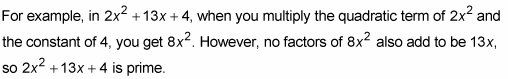##### Pre-Calculus For Dummies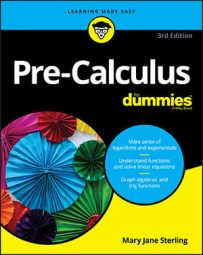In mathematics, factorization or factoring is the breaking apart of a polynomial into a product of other smaller polynomials. If you choose, you could then multiply these factors together, and you should get the original polynomial (this is a great way to check yourself on your factoring skills).

One set of factors, for example, of 24 is 6 and 4 because 6 times 4 = 24. When you have a polynomial, one way of solving it is to factor it into the product of two binomials.

You have multiple factoring options to choose from when solving polynomial equations:

• For a polynomial, no matter how many terms it has, always check for a greatest common factor (GCF) first. Literally, the greatest common factor is the biggest expression that will go into all of the terms. Using the GCF is like doing the distributive property backward.

• If the equation is a trinomial — it has three terms — you can use the FOIL method for multiplying binomials backward.

• If it’s a binomial, look for difference of squares, difference of cubes, or sum of cubes.

Finally, after the polynomial is fully factored, you can use the zero product property to solve the equation.

If a polynomial doesn’t factor, it’s called prime because its only factors are 1 and itself. When you have tried all the factoring tricks in your bag (GCF, backwards FOIL, difference of squares, and so on), and the quadratic equation will not factor, then you can either complete the square or use the quadratic formula to solve the equation. The choice is yours.

You could even potentially choose to always use either completing the square or quadratic formula (and skip the factoring) to solve an equation. Factoring can sometimes be quicker, which is why it is recommended that you try it first.

Standard form for a quadratic expression (simply a quadratic equation without the equal sign) is the x-squared term, followed by the x term, followed by the constant — in other words,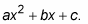If you’re given a quadratic expression that isn’t in standard form, rewrite it in standard form by putting the degrees in descending order. This makes factoring easier (and is sometimes even necessary to factor).

## Always the first step: Look for a GCF

No matter how many terms a polynomial has, it is always important to check for a greatest common factor (GCF) first. If there is a GCF, it will make factoring the polynomial much easier because the number of factors of each term will be lower (because you will have factored one or more of them out!). This is especially important if the GCF includes a variable.

If you forget to factor out this GCF, you may also forget to find a solution, and that could mix you up in more ways than one! Without that solution, you could miss a root, and then you could end up with an incorrect graph for your polynomial.

To factor the polynomial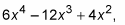1. Break down every term into prime factors.

This expands the expression to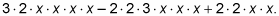2. Look for factors that appear in every single term to determine the GCF.

In this example, you can see one 2 and two x’s in every term. These are underlined in the following: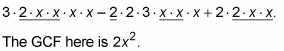3. Factor the GCF out from every term in front of parentheses, and leave the remnants inside the parentheses.

You now have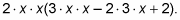4. Multiply out to simplify each term.

This gives you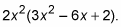5. Distribute to make sure the GCF is correct.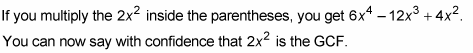## Wrap it up: The FOIL method for trinomials

After you’ve checked a polynomial for a GCF (regardless of whether it had one or not), try to factor again. You may find that it is easier to factor after the GCF has been factored out. The above polynomial had two factors: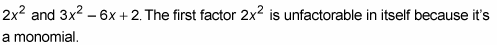However, the second factor may be able to factor again because it’s a trinomial, and if it does you’ll have two more factors that are both binomials.

Most teachers show the guess-and-check method of factoring, where you write down two sets of parentheses —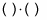— and literally plug in guesses for the factors to see if anything works. Maybe your first guess for this example would be (3x – 2)(x – 1), but if you FOILed this out, you would get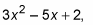and you’d have to guess again. This guess-and-check method is looooooong and tedious, at best. In fact, this particular quadratic is prime, so you could guess and check all day long and it would never factor.

If you’re in pre-calculus and your teacher is using the guess-and-check method of factoring, which just isn’t working for you, you’ve come to the right section. The following procedure, called the FOIL method of factoring (sometimes called the British Method), always works for factoring trinomials and is a very helpful tool if you can’t wrap your brain around guess-and-check. When the FOIL method fails, you know for certain the given quadratic is prime.

The FOIL method of factoring calls for you to follow the steps required to FOIL binomials, only backwards. Remember that when you FOIL, you multiply the First, Outside, Inside, and Last terms together. Then you combine any like terms, which usually come from the multiplication of the Outer and Inner terms.

For example, to factor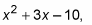1. Check for the GCF first.

The expression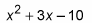won’t have GCF when you break it down and look at it, according to the steps in the last section. The breakdown looks like this: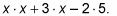No factors that are common to each term, so there is no GCF. That means you get to move onto the next step.

2. Multiply the quadratic term and the constant term.

Be careful of the signs when you do this. In this example, the quadratic term is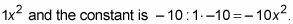3. Write down all the factors of the result, in pairs.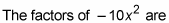• –1x and 10x

• 1x and –10x

• –2x and 5x

• 2x and –5x

4. From this list, find the pair that adds to produce the coefficient of the linear term.

You want the pair whose sum is +3x. For this problem, the answer is –2x and 5x because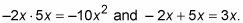5. Break up the linear term into two terms, using the numbers from Step 4 as the coefficients.

Written out, you now have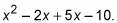It makes life easier in the long run if you always arrange the linear term with the smallest coefficient first. That’s why you put the –2x in front of the +5x.

6. Group the four terms into two sets of two.

Always put a plus sign between the two sets: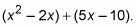7. Find the GCF for each set and factor it out.

Look at the first two terms. What do they share in common? An x. If you factor out the x, you have x(x – 2). Now, look at the second two terms. They share a 5. If you factor out the 5, you have 5(x – 2). The polynomial is now written as x(x – 2) + 5(x – 2).

8. Find the GCF of the two new terms.

Do you see the (x – 2) in both terms? They’re underlined here: x(x – 2) + 5(x – 2). That’s a GCF because it appears in both terms (if you factor using this method, the last step should always look like this). Factor out the GCF from both terms (it’s always the expression inside the parentheses) to the front; you get (x – 2)( ). When you factor it out, the terms that aren’t the GCF are left inside the new parentheses. In this case, you get (x – 2)(x + 5). The (x + 5) is the leftover from taking away the GCF.

Sometimes the sign has to change in Step 6 in order to correctly factor out the GCF. But if you don’t start off with a plus sign between the two sets, you may lose a negative sign you need to factor all the way. For example, in factoring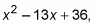you end up in Step 5 with the following polynomial: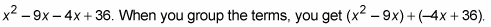Factor out the x in the first set and the 4 in the second set to get x(x – 9) + 4(–x + 9). Notice that the second set is the exact opposite of the first one? In order for you to move to the next step, the sets have to match exactly. To fix this, change the +4 in the middle to –4 and get x(x – 9) – 4(x – 9). Now that they match, you can factor again.

If you follow all the steps in the previous list, you’ll have an easy time with factoring trinomials. Even when an expression has a leading coefficient besides 1, the FOIL method still works. The monkey wrench comes only if there are no factors in Step 2 that add to give you the linear coefficient. In this case, the answer is prime.